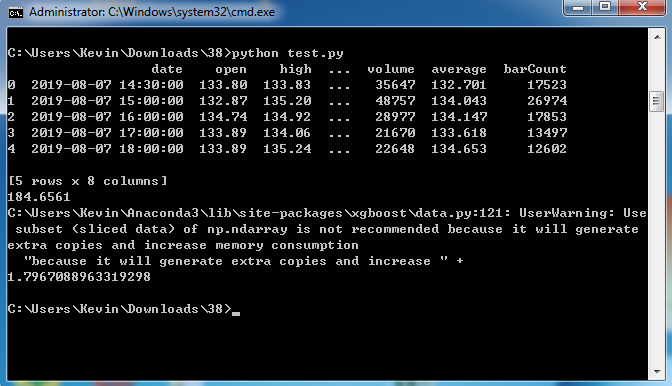Home >  > 利用XGBoost预测股价

## 利用XGBoost预测股价

In this Python tutorial we'll see how we can use XGBoost for Time Series Forecasting, to predict stock market prices with ensemble models.

XGBoost is an optimized distributed gradient boosting library designed to be highly efficient, flexible and portable.

It's basically an ensemble of decision trees where new trees fix errors of the trees that are already part of the model. Trees are added until no further improvements can be made to the model.

The main requirement to use XGBoost for time series is to evaluate the model via walk-forward validation, instead of k-fold cross validation, as k-fold would have biased results.

In this Python tutorial we'll use the XGBRegressor class to make a prediction. XGBRegressor is an implementation of the scikit-learn API for XGBoost regression.（一）RMSE metric 看这里

```from IPython.core.debugger import set_trace

import pandas as pd
import numpy as np
import os
import matplotlib.pyplot as plt
import time

plt.style.use(style="seaborn")

df = df[["close"]].copy()
df["target"] = df.close.shift(-1)
df.dropna(inplace=True)

def train_test_split(data, perc):
data = data.values
n = int(len(data) * (1 - perc))
return data[:n], data[n:]

train, test = train_test_split(df, 0.2)

# print(len(df))
# print(len(train))
# print(len(test))

X = train[:, :-1]
y = train[:, -1]

from xgboost import XGBRegressor

model = XGBRegressor(objective="reg:squarederror", n_estimators=1000)
model.fit(X, y)

val = np.array(test[0, 0]).reshape(1, -1)

pred = model.predict(val)
print(pred)

#Train on train set and predict one sample at a time
def xgb_predict(train, val):
train = np.array(train)
X, y = train[:, :-1], train[:, -1]
model = XGBRegressor(objective="reg:squarederror", n_estimators=1000)
model.fit(X, y)

val = np.array(val).reshape(1, -1)
pred = model.predict(val)
return pred

# print(xgb_predict(train, test[0, 0]))

#Walk-forward validation,We'll evaluate the model with the RMSE metric.
from sklearn.metrics import mean_squared_error

def validate(data, perc):
predictions = []

train, test = train_test_split(data, perc)

history = [x for x in train]

for i in range(len(test)):
test_X, test_y = test[i, :-1], test[i, -1]

pred = xgb_predict(history, test_X)
predictions.append(pred)

history.append(test[i])

error = mean_squared_error(test[:, -1], predictions, squared=False)  #If True returns MSE value, if False returns RMSE value.

return error, test[:, -1], predictions

rmse, y, pred = validate(df, 0.2)

print(rmse)
```